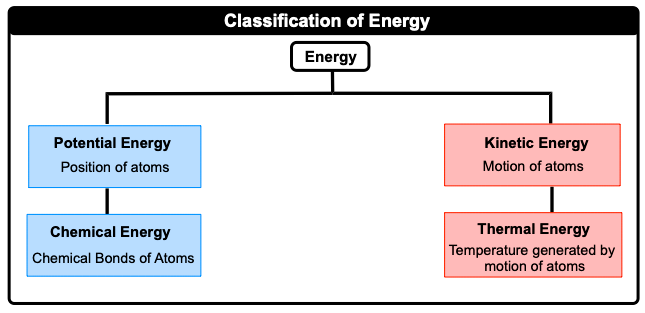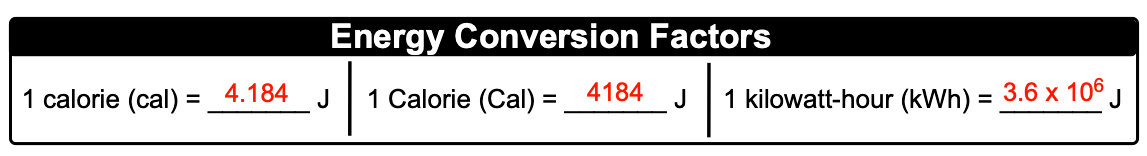Clutch Prep is now a part of Pearson
Ch.6 - Thermochemistry WorksheetSee all chapters

# Nature of Energy

See all sections
Sections
Nature of Energy
Kinetic & Potential Energy
First Law of Thermodynamics
Internal Energy
Endothermic & Exothermic Reactions
Heat Capacity
Constant-Pressure Calorimetry
Constant-Volume Calorimetry
Thermal Equilibrium
Thermochemical Equations
Formation Equations
Enthalpy of Formation
Hess's Law
Enthalpy (IGNORE)

Thermochemistry is the study of matter and energy associated with chemical reactions or physical changes.

###### Classification of Energy

Concept #1: Nature of EnergyConcept #2: Energy Conversion FactorsExample #1: Which of the following statements deals with potential energy with nonchemical energy associated?

Practice: An energy efficient refrigerator uses 780 kWh of electrical energy per year. How many kilocalories of electricity does it use in three years?